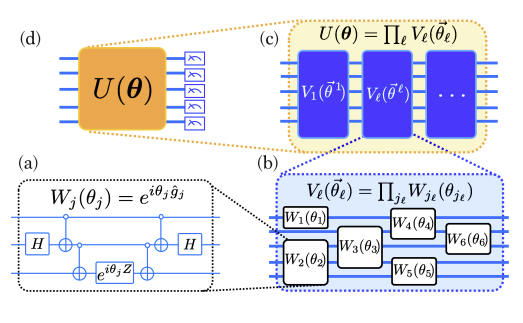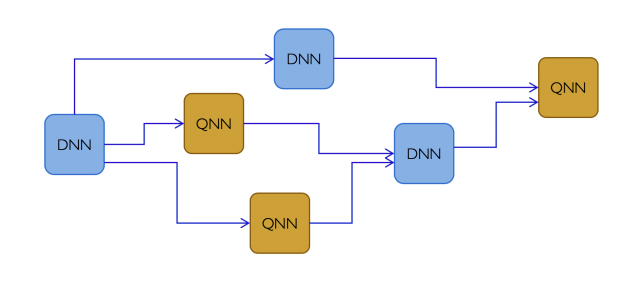# Review- Tensorflow quantum(TFQ) whitepaper — Part2

In this second part, we will look into the fundamental details of the Hybrid Quantum classical machine learning.

Before you get into the details of this article, I would suggest you guys to brush up the fundamentals of quantum vector operations. You can refer to this awesome series on quantum computing to get started with.

# Overview

• In general quantum neural networks can be expressed as the product of the parameterized unitary matrices. Samples i.e. data points or the data distributions and expectation values i.e. you can say as the softmax output are defined by expressing the loss function as an inner product.
• Once we have the QNN defined and the expectation value generated , we can define the gradients then. We finally combine quantum and classical neural networks and formalize hybrid quantum-classical backpropagation.

# Quantum Neural Networks

• Let’s first hypothesis a quantum neural network representation function.

here the Lth layer of the QNN consists of product of V^l i.e a non parametric unitary and U(Θ^l) a unitary with variational parameters. The multi-parameter unitary can comprise of multiple unitaries applied in parallel.
If you don’t understand what a unitary is, you should better refer to the quantum series blogs as mentioned as I mentioned above.

Each of the unitaries can be expressed as the exponential of some generator as shown in the above forumula, which itself can be any Hermitian operator on n qubits. Here P subscript k denotes a Paulis on n-qubits and Beta parameter belongs to real number for all k, j, l.High level view of multi-layer qnn

In diagram (a) we can clearly see that, H is a Hadamard gate which is a constant, non parameterized gate and there is a parameterized gate at the bottom in the 3rd qubit lane.
(b) We see single parameterized gates makes up one unit W(Θ), which makes up the function V(Θ) and the product of these multiple composite functions V(Θ) makes up multiple unitaries Vl that generates the quantum model U(Θ).

# Sampling and Expectations

• To optimize the parameters of an ansatz from equation (1), we need a cost function to optimize. In the case of standard variational quantum algorithms, this cost function is most often chosen to be the expectation value of a cost Hamiltonian, where |Ψ0i is the input state to the parameterized circuit. In general, the cost Hamiltonian can be expressed as a linear combination of operators.

where we defined a vector of coefficients α ∈ R N and a vector of N operators hˆ. Often this decomposition is chosen such that each of these sub-Hamiltonians is in the n-qubit Pauli group hˆ k ∈ Pn.

• Even assuming a perfect fidelity of quantum computation, sampling measurement outcomes of eigenvalues of observables from the output of the quantum computer to estimate an expectation will have some non-negligible variance for any finite number of samples. To get an estimate of the expected value within an accuracy range, one needs to measure samples of the scale of exponents.
• Estimating gradients of quantum neural networks on quantum computers involves the estimation of several expectation values of the cost function for various values of the parameters. One trick that was recently pointed out and has been proven to be successful both theoretically and empirically to estimate such gradients is the stochastic selection of various terms in the quantum expectation estimation. This can greatly reduce the number of measurements needed per gradient update.

# Gradients of Quantum Neural Networks

## Stochastic Parameter Shift Gradient Estimation

• For each component of the gradient vector, for the jth component of the lth layer, the 2Kjl parameter-shifted expectation values to evaluate, thus in total there are 2*KjlMl parameterized expectation values of the cost of the hamiltonian to evaluate.
• As the cost of accurately estimating all these terms one by one and subsequently linearly combining the values such as to yield an estimate of the total gradient may be prohibitively expensive in terms of numbers of runs, instead, one can stochastically estimate this sum, by randomly picking terms according to their weighting.
• In principle, one could go one step further, and per iteration of gradient descent, randomly sample indices representing subsets of parameters for which we will estimate the gradient component, and set the non-sampled indices corresponding gradient components to 0 for the given iteration.
• Doubly stochastic gradient descents
• Triply stochastic gradient descents
• In TFQ, all three of the stochastic averaging methods above can be turned on or off independently for stochastic parameter-shift gradients.

# Hybrid Quantum-Classical Computational GraphsHigh-level depiction of a quantum-classical neural network.
• Hybrid Quantum Classical Neural Networks(HQCNNs) are meta-network based function blocks composed with one another in the topology of a directed graph.
• We can consider this a rendition of a hybrid quantum-classical computational graph where the inner workings (variables, component functions) of various functions are abstracted into boxes. The edges then simply represent the flow of classical information through the metanetwork of quantum and classical functions. The key will be to construct parameterized (differentiable) functions fθ : RM → R N from expectation values of parameterized quantum circuits, then creating a meta-graph of quantum and classical computational nodes from these blocks.
• If we would like the QNN to become more like a classical neural network block, i.e. mapping vectors to vectors f: RM → R N, we can obtain a vector-valued differentiable function from the QNN by considering it as a function of the parameters which outputs a vector of expectation values of different operators.

## Autodifferentiation through hybrid quantum-classical backpropagation

• Here we have classical deep neural networks (DNN) both preceding and postceding the quantum neural network (QNN). e, the preceding DNN outputs a set of parameters θ which are used as then used by the QNN as parameters for inference. The QNN outputs a vector of expectation values (estimated through several runs) whose components are (hθ)k = hhˆkiθ . This vector is then fed as input to another (post-ceding) DNN, and the loss function L is computed from this output. For backpropagation through this hybrid graph, one first backpropagates the gradient of the loss through the post-ceding DNN to obtain gk = ∂L/∂hk. Then, one takes the gradient of the following functions of the output of the QNN: fθ = g·hθ with respect to the QNN parameters θ (which can be achieved with any of the methods for taking gradients of QNN’s described in previous subsections of this section). This completes the backpropagation of gradients of the loss function through the QNN, the preceding DNN can use the now computed ∂L/∂θ to further backpropagate gradients to preceding nodes of the hybrid computational graph.
• By taking gradients of the expectation value of the backpropagated effective Hamiltonian, we can get the gradients of the loss function with respect to QNN parameters, thereby successfully backpropagating gradients through the QNN. Further backpropagation through the preceding function block fpre can be done using standard classical backpropagation by using this evaluated QNN gradient.
• The effective backpropagated Hamiltonian is simply a fixed Hamiltonian operator, as such, taking gradients of the expectation of a single multi-term operator can be achieved by any choice in a multitude of methods for taking gradients of QNN’s described earlier in this section. Backpropagation through parameterized quantum circuits is enabled by our differentiator interface.

--

--